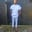Related Tags

dart
communitycreator

# Ternary operator in DartChinweuba Elijah Azubuike

## Overview

The conditional ternary operator assigns a value to a variable based on some conditions. It is used in place of the if statement. This operator also controls the flow of logical execution in the code

Note: The ternary operator is the only dartoperator that takes 3 operators.

### Syntax

condition ? logicIfTrue : logicifFalse

• The condition is the expression whose value determines the used value.
• The value after the ? is returned if the condition returns true.
• The value after the : is returned if the condition returns false.
• ### Example

Let's look at the code below:

import 'dart:convert';
void main() {
int score = 30;
String output = score < 50 ? 'failed': 'Baby';

print(output);
}
Ternary operator in Dart
import 'dart:convert';
void main() {
int score = 95;
String output = score < 45 ? 'Failed'
: score == 45  ? 'Pass'
: 'Distinction';
print(output);
}
Chained ternary operator

### Explanation

In the code above, we try to add grading to the student's score.

• Line 3: We initialize a score variable.
• Line 4: We initialize an output variable which we equate to the ternary operator. We also chain 2 conditions together. The first condition is score < 45 and we use the ? to output the grade if the condition returns true.
• Line 5: We add the second condition using the : then we pass the condition score==45 . And we use the ? to output the grade if the condition returns true.
• Line 6: We use the : to return the default, assuming the 2 condition did not return true.
• Line 7: We print the output variable.

RELATED TAGS

dart
communitycreator

CONTRIBUTORChinweuba Elijah Azubuike
RELATED COURSES

View all Courses

Keep Exploring

Learn in-demand tech skills in half the time